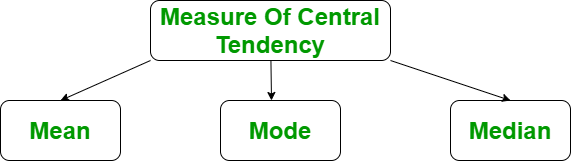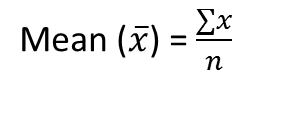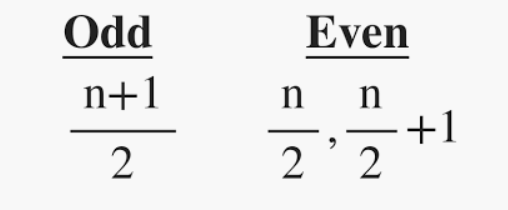Related Articles
Mean, Median and Mode in R Programming
• Last Updated : 22 Jun, 2020

Measure of central tendency in R Language represents the whole set of data by single value. It gives us the location of central points. There are three main measures of central tendency:

• Mean
• Median
• ModePrerequisite:
Before doing any computation, first of all, we need to prepare our data, save our data in an external .txt or .csv files and it’s a best practice to save the file in the current directory. After that import, your data into R as follow:

Get the csv file here.

 `# R program to import data into R ` ` `  `# Import the data using read.csv() ` `myData ``=` `read.csv(``"CardioGoodFitness.csv"``,  ` `                   ``stringsAsFactors ``=` `F) ` `# Print the first 6 rows ` `print``(head(myData)) `

Output:

```   Product Age Gender Education MaritalStatus Usage Fitness Income Miles
1   TM195  18   Male        14        Single     3       4  29562   112
2   TM195  19   Male        15        Single     2       3  31836    75
3   TM195  19 Female        14     Partnered     4       3  30699    66
4   TM195  19   Male        12        Single     3       3  32973    85
5   TM195  20   Male        13     Partnered     4       2  35247    47
6   TM195  20 Female        14     Partnered     3       3  32973    66
```

Functions for computing mean, median and mode:

Analysis R Function
Mean mean()
Median median()
Mode mfv() [modeest]

#### Mean

It is the sum of observation divided by the total number of observations. It is also defined as average which is the sum divided by count.where, n = number of terms

Example:

 `# R program to illustrate ` `# Descriptive Analysis ` ` `  `# Import the data using read.csv() ` `myData ``=` `read.csv(``"CardioGoodFitness.csv"``, ` `                   ``stringsAsFactors ``=` `F) ` ` `  `# Compute the mean value ` `mean ``=` `mean(myData\$Age) ` `print``(mean) `

Output:

``` 28.78889
```

#### Median

It is the middle value of the data set. It splits the data into two halves. If the number of elements in the data set is odd then the center element is median and if it is even then the median would be the average of two central elements.where, n = number of terms

Example:

 `# R program to illustrate ` `# Descriptive Analysis ` ` `  `# Import the data using read.csv() ` `myData ``=` `read.csv(``"CardioGoodFitness.csv"``,  ` `                   ``stringsAsFactors ``=` `F) ` ` `  `# Compute the median value ` `median ``=` `median(myData\$Age) ` `print``(median) `

Output:

``` 26
```

#### Mode

It is the value that has the highest frequency in the given data set. The data set may have no mode if the frequency of all data points is the same. Also, we can have more than one mode if we encounter two or more data points having the same frequency.

Example:

 `# R program to illustrate ` `# Descriptive Analysis ` ` `  `# Import the library ` `library(modeest) ` ` `  `# Import the data using read.csv() ` `myData ``=` `read.csv(``"CardioGoodFitness.csv"``,  ` `                   ``stringsAsFactors ``=` `F) ` ` `  `# Compute the mode value ` `mode ``=` `mfv(myData\$Age) ` `print``(mode) `

Output:

``` 25
```

My Personal Notes arrow_drop_up
Recommended Articles
Page :